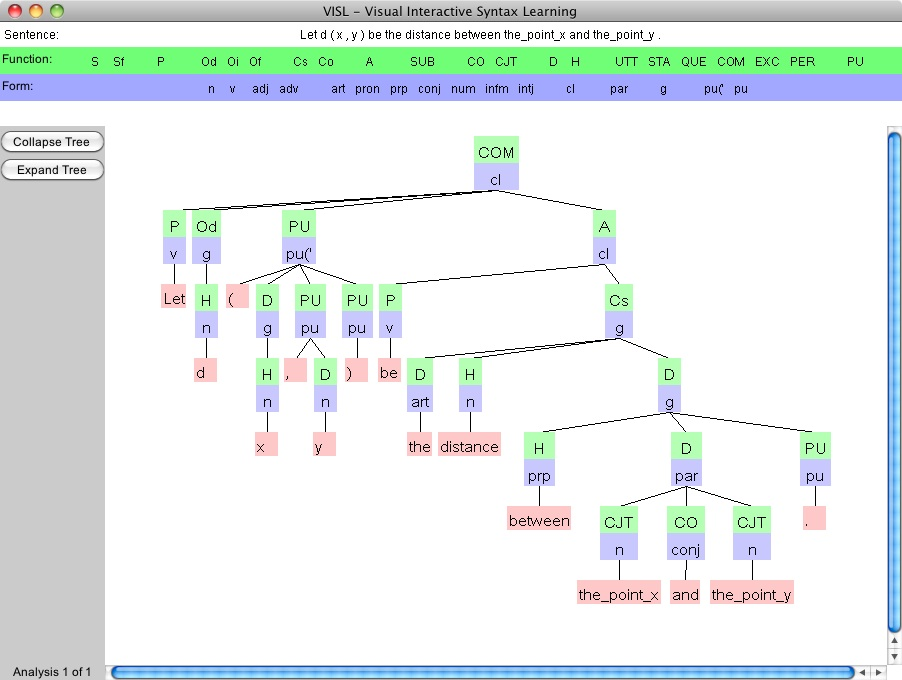# Distance between pointsHere is the result of submitting "Let d(x,y) be the distance..." to the VISL parser.
The parser had difficulty with "distance from x to a", so I rephrased it with "between" plus the conjuction "and" instead.

The notion of distance is studied in mathematics in much the same way as it studies anything else, that is, as something that satisfies some set of rules or axioms and thus suffers the consequences of these rules.
The most general notion of distance belongs to the area of mathematics called metric spaces.
Here, a set M is given with a function d of two variables, each a member of the set M.
The rules are:

1. symmetry, namely d(x, y) = d(y, x), for all x and y in M;
2. identity, namely d(x, y)=0 if and only if x=y, for all x and y in M;
3. and the triangle inequality, namely d(x, y) <= d(x, z) + d(z, y), for all x, y, and z in M.
One of the consequences of being a metric space is having limits of functions.
These functions map from one metric space to another.
Such functions have limits that are defined by the delta epsilon definition presented on the main page of this web site.

Metric spaces are but one step in the process of abstracting the notion of limit.
More generally, the area of mathematics called point set topology provides a setting in which functions have limits without the explicit use of distance.
Here the notion of neighborhood and the notions of open and closed sets prevail and the limit can be defined in terms of neighborhoods.